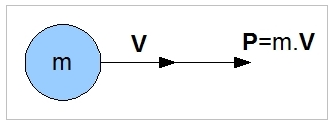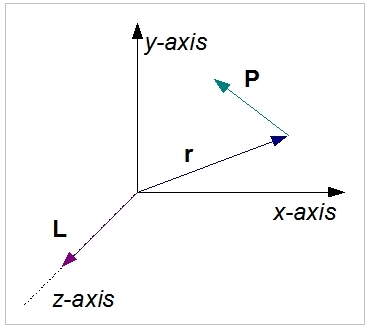# Linear and Angular Momentum

## Linear momentum

The momentum is defined as follows:

P = m . V

where:
P : (linear) Momentum (kg.m/s)
m : Mass (kg)
V : Speed (m/s)

Momentum is the vector product of the mass and speed of a particle.#### Conservation of momentum

Summation [mx . Vx] = C

where:
mx : Massx (kg)
Vx : Speedx (m/s)
C : Constant vector quantity

In a closed system (there are no external forces working on the objects) the total amount of momentum remains constant.

### Explanation

Momentum is the product of the mass and velocity of an object (p = mv). P is a vector quantity.  Momentum is a measure of an object's tendency to move at constant speed along a straight path.

The law of conservation of linear momentum is a fundamental law of nature, and it states that the total momentum of a closed system of objects (which has no interaction with external agents) is constant. This means that it is possible to calculate an unknown velocity following a collision or separation if all the other masses and velocities are known. The line of reasoning used is that the sum of the moments before and after the collision (or separation) is equal.

### Examples

A helicopter is in a hover. The velocity in all directions is 0. The helicopter (3 seater), including pilot and two passengers, has a total weight of 1000 kg. At a particular moment, one of the passengers leaves the helicopter by jumping in a horizontal direction at a speed of 2 m/s. The weight of this passenger is 95 kg. What is the helicopter's speed after the jump?

Before the jump, the sum of all moments is zero (as there is no movement).

mh.vh + mp . vp = 0

Rearranging:

mh.vh = -mp . vp

(1000 - 95) vh = -95 . 2

vh = -190 / 905 = -0,21 m/s

## Angular momentum

Angular momentum of a particle about a given origin is equal to the cross product of position and linear momentum:

L = r x P

where:
L : Angular momentum (N.m.s or kg.m2/s)
r : Position vector relative to the origin
P : The linear momentum of the particle (kg.m/s)

L is a vector quantity. The angular momentum of a system of particles is the sum of that of the particles within it.#### Conservation of Angular Momentum

Summation [rx . Px] = C

where:
rx : Position vectorx (m)
Px : Linear momentumx (m/s)
C : Constant vector quantity

A system's angular momentum stays constant unless an external torque acts on it.

### Explanation

Angular momentum L = r x P is a much used concept in engineering and physics. Angular momentum measures an object's tendency to continue to spin or orbit.
Note that for a rigid body, L can be calculated from L = I . ω.

#### Conservation of Angular Momentum

Angular momentum is important in physics because it is a conserved quantity: a system's angular momentum stays constant unless an external torque acts on it. The conservation of angular momentum explains many phenomena in nature.

The conservation of angular momentum can also apply to non-closed systems. When an object orbits in a force field which is always directed towards some fixed point (the centre), there is then no torque on the body with respect to the centre. This means that the angular momentum of the body about the centre is constant. This situation applies, for example, to the orbit of planets.

The conservation of angular momentum explains the angular acceleration of a rotor blade when it flaps up. As long as the amount of torque applied to the system is constant, the angular momentum remains constant. When a blade flap ups, its centre of mass shifts towards the centre of the rotor system. Therefore, to maintain angular momentum, the angular speed of the blade has to increase.

### Examples

Let’s look at a system that consists of an orbiting mass of 10 kg that rotates about a given axis of rotation. The mass is connected to the centre point with a rod. We assume that the rod has no mass, and is connected at a fixed point on the axis. This point of fixation supports both the horizontal (xy-plane) and vertical (orthogonal to the xy-plane) movements of the rod. The distance from the centre point to the mass is 5 metres.  This system is rotating in the x-y plane at a speed of 500 rpm around the centre point. Then, one of the masses is moved 30 cm upwards in the z-direction. What speed increase will be caused at the corresponding end of the rod?

We notice that the speed vector and the position vector are always at right angles (90 degrees) with each other. Therefore, we get at L = |r| .| P|, which must remain constant.

L = r . m . v = 5 . 10. v
v = r . ω = 5 . (500 . 2π  / 60) = 261.67
L = 13083,33  kg.m2/s

After the upward movement of 30 cm, the length of |r| will not change; however, the effective length for the momentum changes by 5 . cos (α).

α = tan (0.3 / 5)
r = 5 . cos(α)  = 4.991

then, in the situation with the mass moved 30 cm up:

L = r. m . v
13083,33 = 4.991 . 10 . v
v = 13083.33 / 10 / 4.991 =  262.14 m/s

Speed difference = 262.14 - 261.67 = 0.47 m/s.

This result means that the mass of 10 kg at the end of the rod will increase this speed, leading to a forward movement relative to the tip path (path described by the mass) in the plane of rotation.

# Do you want to comment this topic?

1: (Book) Cyclic and Collective2: (Book) Principles of Helicopter Flight4: Logitech Extreme 3D Pro Joystick5: Saitek Pro Flight Rudder Pedals